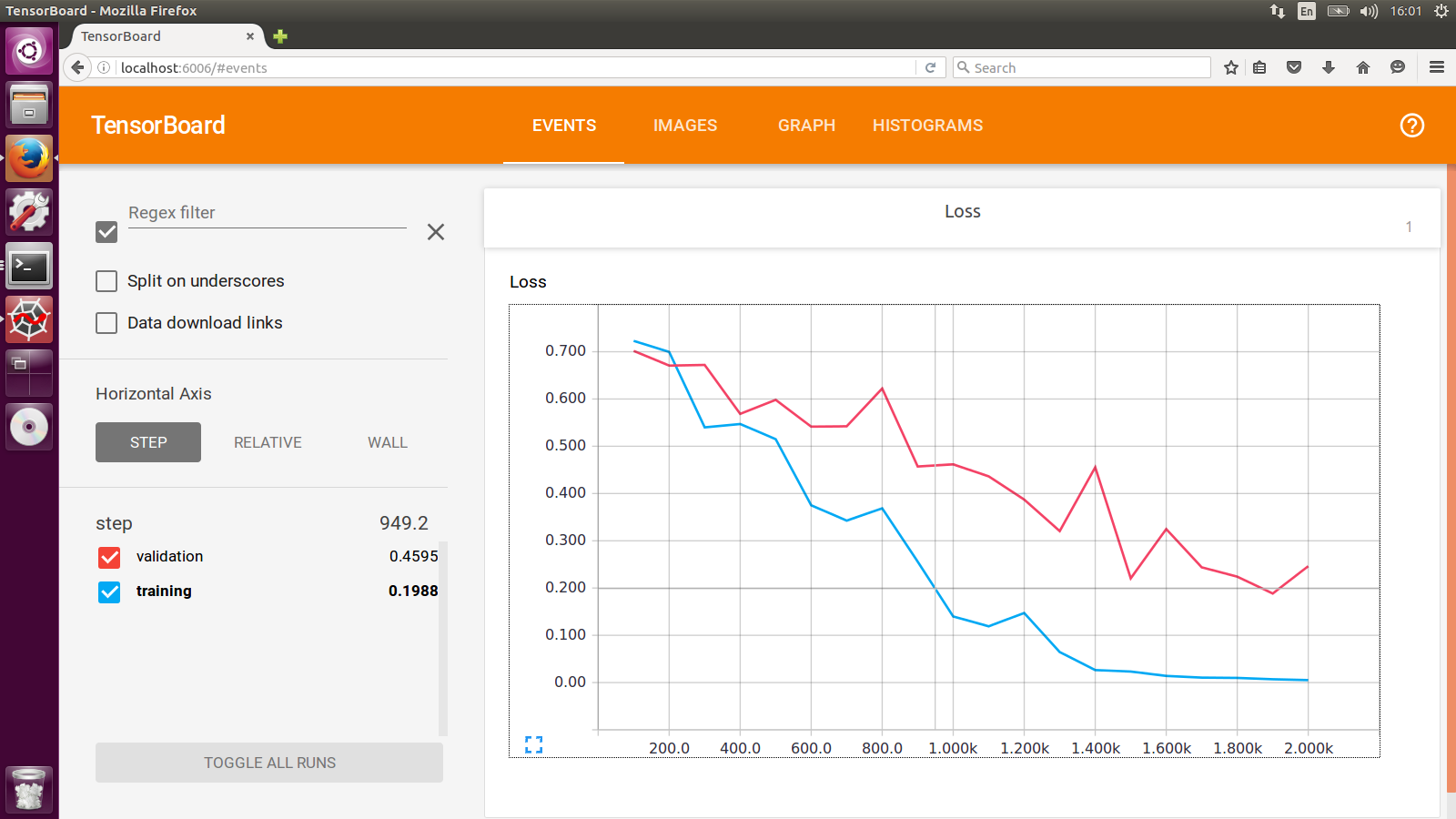2 views

I have trouble understanding the difference (if there is one) between roc_auc_score() and auc() in scikit-learn.

I'm trying to predict a binary output with imbalanced classes (around 1.5% for Y=1).

Classifier

model_logit = LogisticRegression(class_weight='auto')

model_logit.fit(X_train_ridge, Y_train)

Roc curve

false_positive_rate, true_positive_rate, thresholds = roc_curve(Y_test, clf.predict_proba(xtest)[:,1])

AUC's

auc(false_positive_rate, true_positive_rate)

Out: 0.82338034042531527

and

roc_auc_score(Y_test, clf.predict(xtest))

Out: 0.75944737191205602

Somebody can explain this difference? I thought both were just calculating the area under the ROC curve. Might be because of the imbalanced dataset but I could not figure out why.

Thanks!

by (33.1k points)

You can use a summary writer with different log dir for the training set and cross-validation set respectively.

For example: